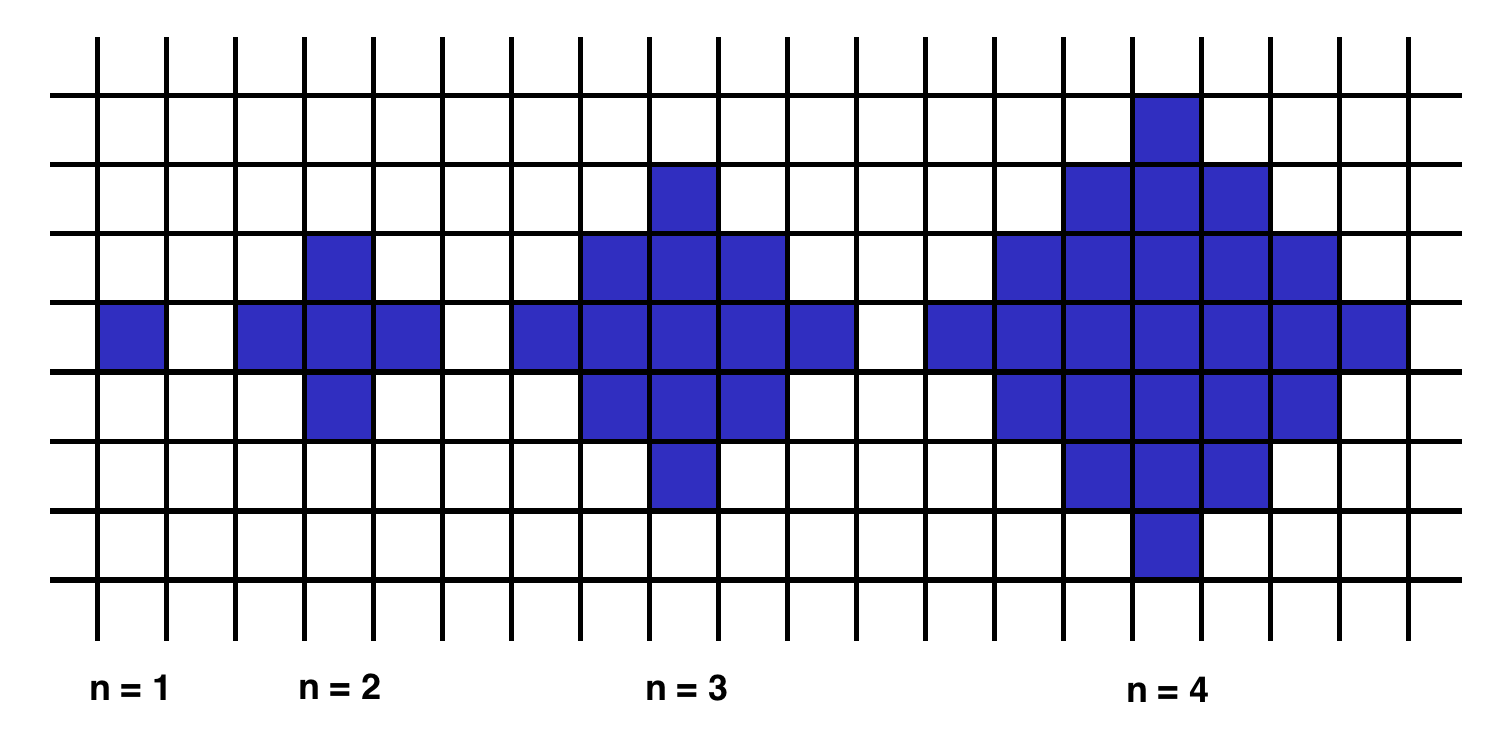## Sunday, April 30, 2017

Below we will define an `n`-interesting polygon. Your task is to find the area of a polygon for a given `n`.
`1`-interesting polygon is just a square with a side of length `1`. An `n`-interesting polygon is obtained by taking the `n - 1`-interesting polygon and appending `1`-interesting polygons to its rim, side by side. You can see the `1`-, `2`-, `3`- and `4`-interesting polygons in the picture below.my solution is

F(1) = 1;
F(n) = F(n-1) + 4(n-1)

Easy

## Monday, April 10, 2017

### Effective Java 규칙12 Comparable 구현을 고려하라

알파벳 순서나 자연적 순서에 따르는 값의 클래스를 구현할때는
Comparable을 구현하는 것을 고려해봐야한다.

고려한다는 말은 추천한다는 말의 의미이지 꼭 써야한다는것은 아니다.

public interface Comparable<T>{
int compareTo(T t);
}

A < B   -1
A == B  0
A > B    1

A<B 이고
B<C 인경우
A<C 이어야한다. 추이성(transitivity)

x.compareTo(y)== 0 이라고 x.equals(y) 가 참은 아니다.

int를 비교할때 대상이 음수라면
comapre로직을 고려해봐야한다.

비교 로직이 a-b > 0  이런식으로 작성할경우

int 의 max 값에서 더 큰수를 더 할 경우  즉
a = 양수 최대값이고
b = 음수면
int범위 밖의 내용에 해당하므로 음수가 반환이 된다.

Comparable은 컬렉션 객체에서 유용하게 쓰인다.